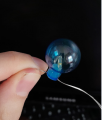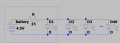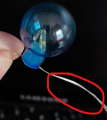# LEDs Series/Parallel circuit

#### kalemaxon89

Joined Oct 12, 2022
91
I have 3 AA 1.5V batteries powering a strip of 40 LEDs that I bought (4.5V total)
I would like to calculate the current and/or voltage on each LED to find out approximately what values we are talking about.

I think the strip of LEDs is in parallel (I attach the photo) but I would like your confirmation.If they are in parallel, I would like to find the current on the first LED.
Drawing the schematic with Vdc, 15 ohm resistor (it's in the box with the batteries) and all the LEDs in parallel I apply ohm's law to the first link (the rectangle you see in this figure)I = (4.5 - Vdiode_forward) / 15

How can I calculate the value of the current on D1 if I do not know the value of its forward voltage ?
Similarly ... how can I calculate the D1 forward voltage if I don't know the value of the current?

I could assume that, normally, a yellow LED supplied with 5V has a Vf=2V ... so from the calculation I would have 166mA of current ... seems a bit too much!

#### Alec_t

Joined Sep 17, 2013
13,337
Can you post a link to the LED strip specification?

#### Jon Chandler

Joined Jun 12, 2008
521
If you measure the voltage drop across the resistor, you can calculate the current being supplied to ALL the LEDs. Divide that current by the number of LEDs to determine the current through each LED.

#### Jerry-Hat-Trick

Joined Aug 31, 2022
232
Unless the LEDs are very well matched putting them in parallel you may see a difference in brightness between them if the circuit is as you have drawn. Ideally, there would be a resistor in series with each LED, each connected between the rails. Let's assume your schematic is correct and the voltage across each LED is 2.0V then the voltage across your 15 ohm resistor with 4.5V supply will be 2.5V. If the resistor is 15 ohms then the total current is indeed about 166mA which, equally divided between the 40 LEDs would be 4.16mA per LED - certainly bright enough for most applications. Power dissipated in the resistor would be 0.42W at 4.5V and 6.7W at 12V which amplifies my thoughts about a single resistor

•kalemaxon89 and djsfantasi

#### kalemaxon89

Joined Oct 12, 2022
91
If you measure the voltage drop across the resistor, you can calculate the current being supplied to ALL the LEDs. Divide that current by the number of LEDs to determine the current through each LED.
I tried to measure the voltage drop on an LED:

I set the voltmeter in the minimum readable range (200mV in this case) but I do not display anything when I try to measure the V at the ends of any LED.
Same thing if I increase the range (if it were out of scale we would display 1).
So maybe I would need a multimeter with more sensitivity and thus a smaller range (maybe 20mV or 2mV)

Is this normal?

#### kalemaxon89

Joined Oct 12, 2022
91
If you measure the voltage drop across the resistor, you can calculate the current being supplied to ALL the LEDs. Divide that current by the number of LEDs to determine the current through each LED.
On the resistor I measured 1V ... so the direct voltage on each LED is 3.5V (4.5V battery) and I=70mA on the resistor.. so 1.75mA on each LED (there are 40 LEDs)

#### djsfantasi

Joined Apr 11, 2010
8,792
Could you explain the picture that you posted? It’s not clear

#### WBahn

Joined Mar 31, 2012
28,192
I tried to measure the voltage drop on an LED:

I set the voltmeter in the minimum readable range (200mV in this case) but I do not display anything when I try to measure the V at the ends of any LED.
Same thing if I increase the range (if it were out of scale we would display 1).
So maybe I would need a multimeter with more sensitivity and thus a smaller range (maybe 20mV or 2mV)

Is this normal?
What do you mean that you measured the voltage at the ends of any LED?

You want to measure the voltage ACROSS the LED. Put one lead (the red lead, most likely) at the junction between the resistor and the LEDs and the other lead (the black one, most likely) at the other end of the LED (if they truly are in parallel, that will be the negative terminal of the battery).

It sounds like you are measuring the voltage across a piece of wire going from the cathode of the LED to the battery.

#### kalemaxon89

Joined Oct 12, 2022
91
Can you post a link to the LED strip specification?
Unfortunately it is not in the box that came to me

#### WBahn

Joined Mar 31, 2012
28,192
On the resistor I measured 1V ... so the direct voltage on each LED is 3.5V (4.5V battery) and I=70mA on the resistor.. so 1.75mA on each LED (there are 40 LEDs)
Is that 1 V the voltage across the resistor? I.e., one lead on one side of the resistor and the other lead on the other side of the resistor?

If so, 1.75 mA per LED (I get 1.67 mA, assuming a 15 Ω resistor, but close enough) is reasonable for some types of LEDs.

#### kalemaxon89

Joined Oct 12, 2022
91
Is that 1 V the voltage across the resistor? I.e., one lead on one side of the resistor and the other lead on the other side of the resistor?

If so, 1.75 mA per LED (I get 1.67 mA, assuming a 15 Ω resistor, but close enough) is reasonable for some types of LEDs.
Yes it's 1V.
The calculations are correct .. but I don't understand why the multimeter do not display the voltage when I measure it across the LEDs.
Maybe because the cables could be shielded? (to avoid shocks given when you touch them directly with your hands ... although we are talking about such small currents that there should be no danger)

@Jerry-Hat-Trick

#### Audioguru again

Joined Oct 21, 2019
5,595
I have a cheap flashlight with 60 surface mount LEDs on one side. They are all in parallel and connected directly to three AAA battery cells in series with no resistor. The original battery was poor quality "super-heavy-duty" cells that did not last long so I replaced it with three AAA Name Brand alkaline cells and Oh My Goodness it is extremely bright!

#### Attachments

Last edited:
•kalemaxon89

#### Kjeldgaard

Joined Apr 7, 2016
476
•djsfantasi

#### djsfantasi

Joined Apr 11, 2010
8,792
Slightly enlarged and cropped image:

View attachment 283387
It looks like Chip LEDs soldered directly on a very thin two-wire solderable wire, so it is difficult to measure the LED voltage directly on the individual balls, without destroying the light chain.
Where is the resistor?

#### Kjeldgaard

Joined Apr 7, 2016
476
Where is the resistor?
From the starting thread: "Drawing the schematic with Vdc, 15 ohm resistor (it's in the box with the batteries)"

#### WBahn

Joined Mar 31, 2012
28,192
Slightly enlarged and cropped image:

View attachment 283387
It looks like Chip LEDs soldered directly on a very thin two-wire solderable wire, so it is difficult to measure the LED voltage directly on the individual balls, without destroying the light chain.
In the original image, it looks like there are two wires exiting the bulb to the right, but I can't tell about the wire he is holding. Is that also two wires? Doesn't look like it, but I can't rule it out. If they are all in parallel, it would have to be.

#### Kjeldgaard

Joined Apr 7, 2016
476
@WBahn

The wire apparently runs unbroken through the ball, but with the two wires separated to connect to the LED.

I cannot determine whether it is a solderable, perhaps varnish-like insulation on the wire.

#### kalemaxon89

Joined Oct 12, 2022
91
Slightly enlarged and cropped image:

View attachment 283387
It looks like Chip LEDs soldered directly on a very thin two-wire solderable wire, so it is difficult to measure the LED voltage directly on the individual balls, without destroying the light chain.
I'm sorry for the few informations shared.
I hope to provide some clarification:

In the box containing the battery (let's assume it is one and not three in series) two wires come out: one comes directly from the - of the battery while the other comes from the + of the battery and it's connected to the 15 ohm resistor (so the resistor is in series with the + of the battery).
These two wires (I indicate them in red in the photo below) are connected to the two ends of the first LED (you can see it in the photo).
The other end of the LED connects to the next LED and so on until the 40th LED.
It looks like a parallel connection to me because one end of each led is connected to the + of the battery .. while the others ends (of each LED) are connected to the - .. so it doesn't look like a series connection to meLast edited:

#### BobTPH

Joined Jun 5, 2013
6,317
On the resistor I measured 1V ... so the direct voltage on each LED is 3.5V (4.5V battery) and I=70mA on the resistor.. so 1.75mA on each LED (there are 40 LEDs)
Don’t assume the battery voltage is 4.5V. Measure it with the lights on.

And sorry if I missed it, but what color are the LEDs?

#### WBahn

Joined Mar 31, 2012
28,192
I'm sorry for the few informations shared.
I hope to provide some clarification:

In the box containing the battery (let's assume it is one and not three in series) two wires come out: one comes directly from the - of the battery while the other comes from the + of the battery and it's connected to the 15 ohm resistor (so the resistor is in series with the + of the battery).
These two wires (I indicate them in red in the photo below) are connected to the two ends of the first LED (you can see it in the photo).
The other end of the LED connects to the next LED and so on until the 40th LED.
It looks like a parallel connection to me because one end of each led is connected to the + of the battery .. while the others ends (of each LED) are connected to the - .. so it doesn't look like a series connection to me

View attachment 283390
It sounds like they are in parallel, but could you please confirm that the wire you are holding in your hand on the left is actually two wires?